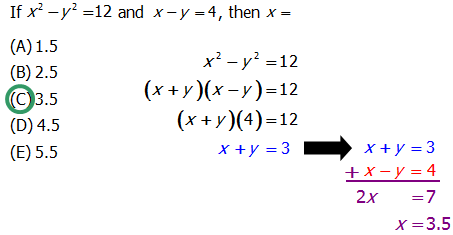# Quant: Difference of Two Squares

## Prime numbers

You may remember this formula, one of the sleekest factoring tricks in all of algebra:

\bold{y^2 - x^2 = (y + x)(y - x)}

This formula, called “the difference of two squares” formula, is a favorite of standardized test writers. A simple enough pattern: see if you can detect where it shows up in the following challenging problems.

Q1. \dfrac{1}{2 - \sqrt{3}}=

(A) 2 - \sqrt{3}

(B) 2 + \sqrt{3}

(C) \dfrac{1}{7}

(D) \dfrac{1}{2} - \dfrac{1}{\sqrt{3}}

(E) \dfrac{1}{2} + \dfrac{1}{\sqrt{3}}

Q2. What is the sum of a and b?

Statement 1: a = 4

Statement 2: \dfrac{b^2 - a^2}{b - a} = 7

(A) Statement 1 alone is sufficient but statement 2 alone is not sufficient to answer the question asked.

(B) Statement 2 alone is sufficient but statement 1 alone is not sufficient to answer the question asked.

(C) Both statements 1 and 2 together are sufficient to answer the question but neither statement is sufficient alone.

(D) Each statement alone is sufficient to answer the question.

Q3. In the diagram above, \angle{A} = \angle{ABC}, \angle{CBD} = \angle{BDC}, and \angle{CBE} = 90\degree. If AE = 16 and DE = 4, what is the length of BE?

(A) 7

(B) 8

(C) 9

(D) 10

(E) 11

Q4. If x^2 - y^2 = 12 and x - y = 4, then x =

(A) 1.5

(B) 2.5

(C) 3.5

(D) 4.5

(E) 5.5

## Practice Problem Solutions

Q1. This involves a relatively sophisticated trick known as “multiplying by the conjugate.” When we have an expression of the form a + \sqrt{b}, the “conjugate” of this is a - \sqrt{b}. When we multiply a radical expression by its conjugate, we employ the difference of two squares. For example:

\dfrac{1}{2 - \sqrt{3}} = \dfrac{1}{2 - \sqrt{3}} \times \dfrac{2 + \sqrt{3}}{2 + \sqrt{3}} = \dfrac{2 + \sqrt{3}}{2^2 - (\sqrt{3})^2} = \dfrac{2 + \sqrt{3}}{4 - 3} = \dfrac{2 + \sqrt{3}}{1} = 2 + \sqrt{3}

This is answer B. BTW, the trick of multiplying by the conjugate is at the very outside edge of what you might be expected to do the hardest QUANT math problems.

Q2. The prompt of this DS problem is straightforward.

Statement #1 tells us a = 4, but we have no idea of b, so, by itself, this is not sufficient for finding the sum.

Statement #2 gives us a value for an algebraic expression that lends itself nicely to simplification.

7 = \dfrac{b^2 - a^2}{b - a} = \dfrac{(b-a)(b+a)}{b-a} = a + b

Thus, the sum is 7. Statement #2, by itself is sufficient.

Q3. This is a tricky one. Remember, it’s a diagram drawn to scale, so if all else fails, you can estimate (see this post). But, let’s solve this with math. The fact that \angle{A} = \angle{ABC} tells us \triangle{ABC} is isosceles, with AC = BC. The fact that \angle{CBD} = \angle{BDC} tells us \triangle{BCD} is isosceles, with BC = CD. The fact that \angle{CBE} = 90\degree means that (BC)^2 + (BE)^2 = (CE)^2. This means

(BE)^2 = (CE)^2 - (BC)^2

= (CE + BC)(CE - BC)

= (CE + AC)(CE - CD) (substitutions from the two isosceles triangles)

= (AE)(DE)

= (16)(4) = 64

so

BE = 8